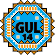General Utility Library for C++14  2.9
gul14/bit_manip.h

## Detailed Description

Bit manipulation.

## Typedefs

template<typename T >
using gul14::BitFunctionReturnType = std::enable_if_t< std::is_integral< T >::value and not std::is_same< std::decay_t< T >, bool >::value, std::decay_t< T > >
Return type of the bit manipulation functions. More...

## Functions

template<typename T = unsigned, typename ReturnT = BitFunctionReturnType<T>>
constexpr auto gul14::bit_set (unsigned bit) noexcept -> ReturnT
Set a bit in an integral type. More...

template<typename T , typename ReturnT = BitFunctionReturnType<T>>
constexpr auto gul14::bit_set (T previous, unsigned bit) noexcept -> ReturnT
Set a bit in an integral value. More...

template<typename T , typename ReturnT = BitFunctionReturnType<T>>
constexpr auto gul14::bit_reset (T previous, unsigned bit) noexcept -> ReturnT
Reset a bit in an integral value. More...

template<typename T , typename ReturnT = BitFunctionReturnType<T>>
constexpr auto gul14::bit_flip (T previous, unsigned bit) noexcept -> ReturnT
Flip a bit in an integral value. More...

template<typename T >
constexpr bool gul14::bit_test (T bits, unsigned bit) noexcept
Test a bit in an integral value. More...

## ◆ BitFunctionReturnType

template<typename T >
 using gul14::BitFunctionReturnType = typedef std::enable_if_t< std::is_integral::value and not std::is_same, bool>::value, std::decay_t >

Return type of the bit manipulation functions.

We want to SFINAE out of all types except integers. Because bools counts as integer type and we don't want that we specifically exclude it here.

The type is then simply the decayed input type, to get rid of const or volatile specifiers.

Template Parameters
 T Type specified/deduced by bit_*() user

## ◆ bit_flip()

template<typename T , typename ReturnT = BitFunctionReturnType<T>>
 constexpr auto gul14::bit_flip ( T previous, unsigned bit ) -> ReturnT
inlineconstexprnoexcept

Flip a bit in an integral value.

Flips the bit number bit in the existing value previous. This inverts the state of the bit: setting the bit if it was previously not set and resetting the bit if it was previously set.

When bit is greater or equal to the number of bits in type T, std::abort() is called (via assert()).

Parameters
 previous Existing integral value where the bit shall be modified bit Number of the bit that is to be modified (LSB == 0)
Returns
New integral value with the bit modified.
Template Parameters
 T Type of the bit-holding integral value.
Since
GUL version 1.8
bit_set(bit), bit_set(previous, bit), bit_reset(previous, bit), bit_test(bits, bit)

## ◆ bit_reset()

template<typename T , typename ReturnT = BitFunctionReturnType<T>>
 constexpr auto gul14::bit_reset ( T previous, unsigned bit ) -> ReturnT
inlineconstexprnoexcept

Reset a bit in an integral value.

Reset (clear) the bit number bit in the existing value previous.

When bit is greater or equal to the number of bits in type T, std::abort() is called (via assert()).

Parameters
 previous Existing integral value where the bit shall be modified bit Number of the bit that is to be modified (LSB == 0)
Returns
New integral value with the bit modified.
Template Parameters
 T Type of the bit-holding integral value.
Since
GUL version 1.8
bit_set(bit), bit_set(previous, bit), bit_flip(previous, bit), bit_test(bits, bit)

## ◆ bit_set() [1/2]

template<typename T , typename ReturnT = BitFunctionReturnType<T>>
 constexpr auto gul14::bit_set ( T previous, unsigned bit ) -> ReturnT
inlineconstexprnoexcept

Set a bit in an integral value.

Set the bit number bit in the existing value previous.

When bit is greater or equal to the number of bits in type T, std::abort() is called (via assert()).

Parameters
 previous Existing integral value where the bit shall be modified bit Number of the bit that is to be modified (LSB == 0)
Returns
New integral value with the bit modified.
Template Parameters
 T Type of the bit-holding integral value.
Since
GUL version 1.8
bit_set(bit), bit_reset(previous, bit), bit_flip(previous, bit), bit_test(bits, bit)

## ◆ bit_set() [2/2]

template<typename T = unsigned, typename ReturnT = BitFunctionReturnType<T>>
 constexpr auto gul14::bit_set ( unsigned bit ) -> ReturnT
inlineconstexprnoexcept

Set a bit in an integral type.

Return an integral value of type T where the bit number bit and only that bit is set.

When bit is greater or equal to the number of bits in type T, std::abort() is called (via assert()).

Parameters
 bit Number of the bit that is to be set (LSB == 0)
Returns
Integral value with the bit set.
Template Parameters
 T Type of the bit-holding integral value.
Note
If no type T is specified an unsigned int will be returned.
Since
GUL version 1.8
bit_set(previous, bit), bit_reset(previous, bit), bit_flip(previous, bit), bit_test(bits, bit)

## ◆ bit_test()

template<typename T >
 constexpr bool gul14::bit_test ( T bits, unsigned bit )
inlineconstexprnoexcept

Test a bit in an integral value.

Test the bit number bit in the existing value bits.

When bit is greater or equal to the number of bits in type T, std::abort() is called (via assert()).

Parameters
 bits Integral value where the bit shall be tested bit Number of the bit that is to be modified (LSB == 0)
Returns
True or false
Template Parameters
 T Type of the bit-holding integral value.
Since
GUL version 1.8